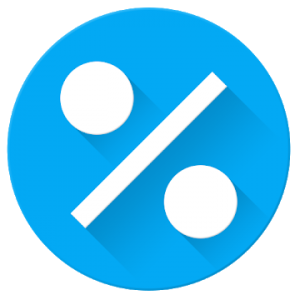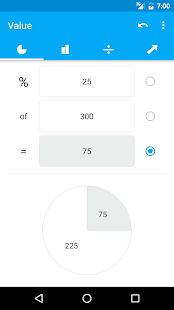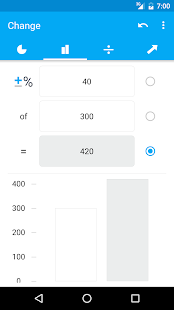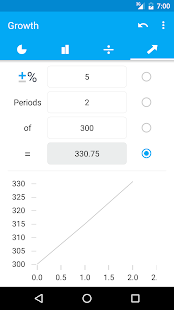Percentage Calculator is simply the fastest way to calculate discounts, tips, interest and other percentages.

Instantly calculate and visualize all kind of percentages. Any input value can either be a source or result of a calculation — just enter the values you know and it will calculate the remaining one.

The supported calculators and their possible use cases of this Percentage Calculator are:

• Value: (Sales Tax, VAT, School (mathematics, statistics, algebra), Fat Percentage in Food, Cooking Ingredients)
• Change: (Percentage Increase/Decrease, Tips, Markups, Profit Margin, Shopping Discounts, Sale Price, Net/Gross Price; Return on Investment (ROI)
• Conversiom: (Convert Fractions)
• Growth: (Business and Financial, Compound Interest, Inflation, Cumulative Growth, Compound Annual Growth Rate (CAGR), Doubling Time (Rule of 72))

What’s New
In this update, we’ve improved app performance and reduced the storage size.
If you like our app we would be happy if you could leave a ★★★★★ rating.

••••